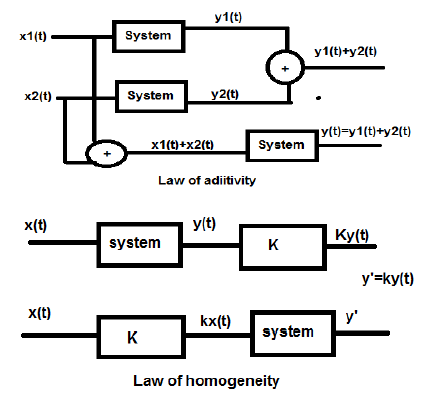# Digital Signal Processing - Linear Systems

#### Digital Marketing Online Training

23 Lectures 2 hours

#### How To Start Your Own Digital Marketing Agency

125 Lectures 9 hours

#### Digital Marketing Agency Elite Consultants Masterclass

140 Lectures 20 hours

A linear system follows the laws of superposition. This law is necessary and sufficient condition to prove the linearity of the system. Apart from this, the system is a combination of two types of laws −

• Law of additivity
• Law of homogeneityBoth, the law of homogeneity and the law of additivity are shown in the above figures. However, there are some other conditions to check whether the system is linear or not.

The conditions are

• The output should be zero for zero input.
• There should not be any non-linear operator present in the system.

Examples of non-linear operators −

(a) Trigonometric operators- Sin, Cos, Tan, Cot, Sec, Cosec etc.

(b) Exponential, logarithmic, modulus, square, Cube etc.

(c) sa(i/p) , Sinc (i/p) , Sqn (i/p) etc.

Either input x or output y should not have these non-linear operators.

## Examples

Let us find out whether the following systems are linear.

a) $y(t) = x(t)+3$

This system is not a linear system because it violates the first condition. If we put input as zero, making x(t) = 0, then the output is not zero.

b) $y(t) = \sin tx(t)$

In this system, if we give input as zero, the output will become zero. Hence, the first condition is clearly satisfied. Again, there is no non-linear operator that has been applied on x(t). Hence, second condition is also satisfied. Therefore, the system is a linear system.

c) $y(t) = \sin (x(t))$

In the above system, first condition is satisfied because if we put x(t) = 0, the output will also be sin(0) = 0. However, the second condition is not satisfied, as there is a non-linear operator which operates x(t). Hence, the system is not linear.